## Cosine Angle between Two Vectors

Cosine angle between two vectors is equal to their dot product divided by the product of their norms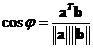.

Example:
Suppose we have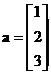and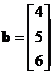, the vector inner product is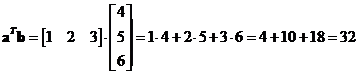Their norms are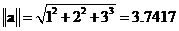and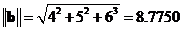.
Therefore, the cosine angle is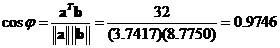and the angle is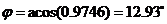.

The interactive program below produces cosine angle between two vectors of the same dimension and also specify the angle in both radians and degrees. Random Example button will generate random vectors at the right format.

vector x vector y

## Properties

Some important properties of related to cosine angle are

• Two vectors are orthogonal if their dot product is zero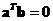, which means the cosine angle is zero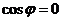• Two vectors are parallel if the absolute value of their dot product is equal to the product of their norms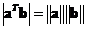, which means the absolute cosine angle is one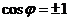.
• Two vectors are in the same direction if their dot product is equal to the product of their norms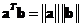, which mean the cosine angle is exactly one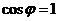.
• Since the value of Cosine is between -1 and +1, the absolute value of vector dot product is always less than or equal to the product of their norms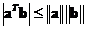. This is called Cauchy-Schwartz inequality.